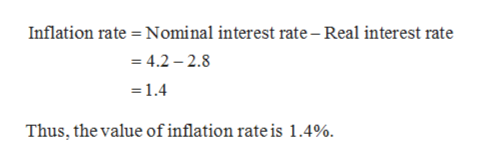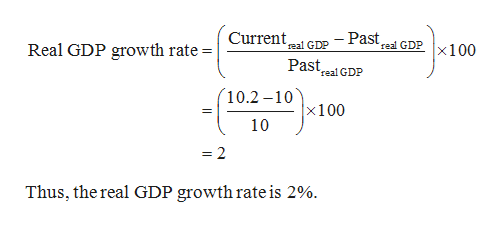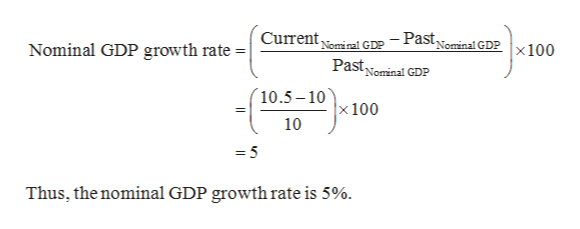The growth rate of real GDP is 6.4%The growth rate of nominal GDP is 7.8%The nominal interest rate is 4.2%The real interest rate is 2.8%The money supply​ (M2) is \$11,438(in billions) Use the information given above to calculate the inflation rate. According to the quantity theory of​ money, if in a​ year's time, real GDP grew from​ \$10 trillion to​ \$10.2 trillion, and nominal GDP for the same time period grew from​ \$10 trillion to​ \$10.5 trillion, what is the growth rate of money supply? And the inflation rate?

Question

The growth rate of real GDP is 6.4%

The growth rate of nominal GDP is 7.8%

The nominal interest rate is 4.2%

The real interest rate is 2.8%

The money supply​ (M2) is \$11,438(in billions)

Use the information given above to calculate the inflation rate.

• According to the quantity theory of​ money, if in a​ year's time, real GDP grew from​ \$10 trillion to​ \$10.2 trillion, and nominal GDP for the same time period grew from​ \$10 trillion to​ \$10.5 trillion, what is the growth rate of money supply? And the inflation rate?
Step 1

According to the quantity theory of money, inflation rate can be calculated as follows:help_outlineImage TranscriptioncloseInflation rate Nominal interest rate - Real interest rate = 4.2 2.8 =1.4 Thus, the value of inflation rate is 1.4%. fullscreen
Step 2

The real GDP growth rate:help_outlineImage TranscriptioncloseCurrenteal GDP -Past GDPX100 Real GDP growth rate = PastalGDP rea 10.2 -10 x100 10 = 2 Thus, the real GDP growth rate is 2% fullscreen
Step 3

The nominal GDP gr...help_outlineImage TranscriptioncloseCurrentminal GDP - Pastominal GDPx100 Pastominal GDP Nominal GDP growth rate | N 10.5-10 x 100 10 Thus, the nominal GDP growth rate is 5%. fullscreen

Want to see the full answer?

See Solution

Want to see this answer and more?

Our solutions are written by experts, many with advanced degrees, and available 24/7

See Solution
Tagged in